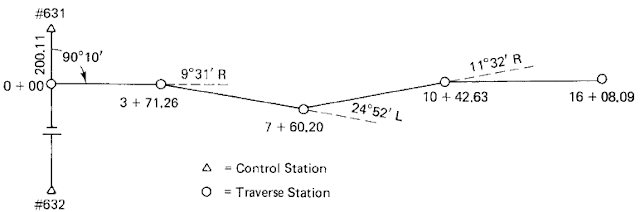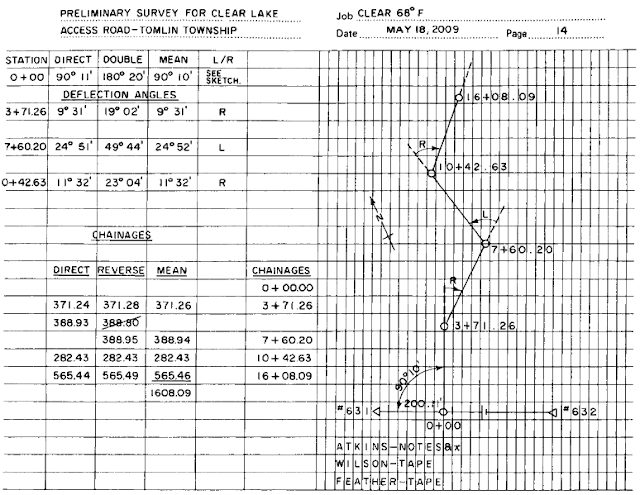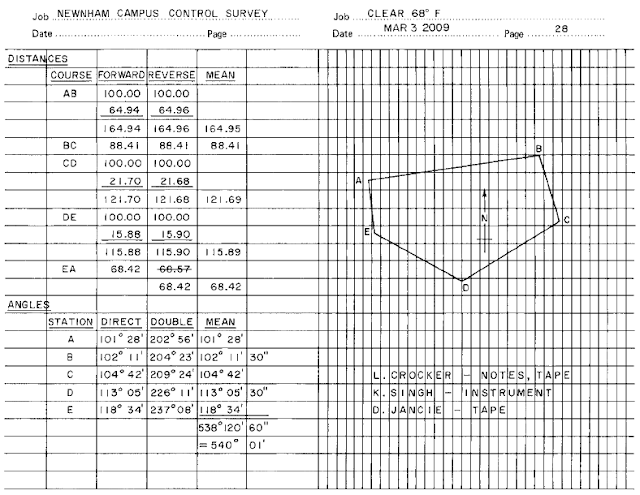# Overview: Open and Closed Traverses in Surveying

### 1. Background

A traverse is a form of control survey used in a wide variety of engineering and property surveys. Essentially, traverses are a series of established stations tied together by angle and distance. Angles are measured by theodolites or total stations; the distances can be measured by electronic distance measurement (EDM) instruments, sometimes by steel tapes. Traverses can be open, as in route surveys, or closed, as in closed geometric figures (Figures 1 and 2). Traverse surveying has been one of the most basic survey types being used for ages by surveyors. Closed traverse survey using total station has particularly become popular in recent times. It is however pivotal to understand the underlying concepts of open traverse calculations and closed traverse calculations.Figure 1: Open traverse
Traverse computations are used to do the following: balance field angles, compute latitudes and departures, compute traverse error, distribute the errors by balancing the latitudes and departures, adjust original distances and directions, compute coordinates of the traverse stations, and compute the area enclosed by a closed traverse. In modern practice, these computations are routinely performed on computers and/or on some total stations or their electronic field books/data collectors. In this article, we will perform traverse computations manually (using calculators) to demonstrate and reinforce the mathematical concepts underlying each stage of these computations.Figure 2: Closed traverse or loop traverse

In engineering work, traverses are used as control surveys
(1) to locate topographic detail for the preparation of topographic plans and engineering design plan and profiles,
(2) to lay out (locate) engineering works, and
(3) for the processing and ordering of earthwork and other engineering quantities. Traverses can also help provide horizontal control for aerial surveys in the preparation of photogrammetric mapping.

### 1.1 Open Traverse

An open traverse (Figure 1) is particularly useful as a control for preliminary and construction surveys for highways, roads, pipelines, electricity transmission lines, and the like. These surveys may be from a few hundred feet (meters) to many miles (kilometers) in length. The distances are normally measured by using EDM (sometimes steel tapes). Each time the survey line changes direction, a deflection angle is measured with a theodolite or total station. Deflection angles are measured from the prolongation of the back line to the forward line (Figure 1); the angles are measured either to the right or to the left (L or R), and the direction (L or R) is shown in the field notes, along with the numerical values.Figure 3: Field notes for open traverse
Angles are measured at least twice to eliminate mistakes and to improve accuracy. The distances are shown in the form of stations (chainages), which are cumulative measurements referenced to the initial point of the survey, 0 + 00. See Figure 3 for typical field notes for a route survey. Open traverses may extend for long distances without the opportunity for checking the accuracy of the ongoing work. Thus, all survey measurements are repeated carefully at the time of the work, and every opportunity for checking for position and direction is utilized (adjacent property surveys and intersecting road and railroad rights-of-way are checked when practical.) Global positioning system (GPS) surveying techniques are also used to determine and verify traverse station positioning.

Many states and provinces have provided densely placed control monuments as an extension to their coordinate grid systems. It is now possible to tie in the initial and terminal survey stations of a route survey to coordinate control monuments. Because the Y and X (and Z) coordinates of these monuments have been precisely determined, the route survey changes from an open traverse to a closed traverse and is then subject to geometric verification and analysis. Of course, it is now also possible, using appropriate satellite-positioning techniques, to directly determine the easting, northing, and elevation of all survey stations.

### 1.2 Closed Traverse

A closed traverse is one that either begins and ends at the same point or begins and ends at points whose positions have been previously determined (as described above). In both cases, the angles can be closed geometrically, and the position closure can be determined mathematically. A closed traverse that begins and ends at the same point is called a loop traverse (Figure 2). In this case, the distances are measured from one station to the next and verified, using a steel tape or EDM instrument. The interior angle is measured at each station, and each angle is measured at least twice. Figure 4 illustrates typical field notes for a loop traverse survey. In this type of survey, distances are booked simply as dimensions, not as stations or chainages.Figure 3: Field notes for open traverse
[blogger]

Engineeersdaily

Name

Email *

Message *